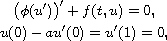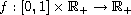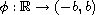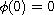Electron. J. Diff. Equ., Vol. 2016 (2016), No. 51, pp. 1-8.

### Positive solutions for second-order boundary-value problems with phi-Laplacian Diana-Raluca Herlea

Abstract:
This article concerns the existence, localization and multiplicity of positive solutions for the boundary-value problemwhereis a continuous function andis an increasing homeomorphism with. We obtain existence, localization and multiplicity results of positive solutions using Krasnosel'skii fixed point theorem in cones, and a weak Harnack type inequality. Concerning systems, the localization is established by the vector version of Krasnosel'skii theorem, where the compression-expansion conditions are expressed on components.

Submitted February 3, 2016. Published February 18, 2016.
Math Subject Classifications: 34B18, 47H10.
Key Words: Positive solution; phi-Laplacian, boundary value problem; Krasnosel'skii fixed point theorem; weak Harnack inequality.

An addendum was posted on May 19, 2016. It gives an additional hypothesis for Theorem 2.2, and gives examples for Theorems 2.4 and 2.5. See the last four pages of this article.

Show me the PDF file (207 KB), TEX file for this article.Diana-Raluca Herlea Babes-Bolyai University Department of Mathematics 400084 Cluj, Romania email: dherlea@math.ubbcluj.ro